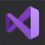# Harmonic progression

Suppose there is a $1m$ rubber band with an ant on it. Every time the ant walks $1cm$, the rubber band expands and the circumference increases by $1m$, and the length of the ant from the starting point also becomes longer. So, can the ants complete a circle? I think it can't do it, but it can. First, it takes $1\%$ of the entire rubber band. Then, it went $0.5\%$. Then, $0.333\%$, $0.25\%$, $0.2\%$... Add them up: $\frac{1}{100}+\frac{\frac{1}{2}}{100}+\frac{\frac{1}{3}}{100}+\cdots$ $= \frac{1}{100} \times (\color{#D61F06}{1+\frac{1}{2}+\frac{1}{3}+\cdots})$ It is a harmonic series, and the result is positive infinity. But we don't need positive infinity, we just need to make it greater than 100. Let $1+\frac{1}{2}+\frac{1}{3}+\cdots+\frac{1}{a} = 100$ $\int_{0}^{1}{(1+x+x^2+x^3+\cdots+x^{a-1}) \ dx = 100}$ $\int_0^1 {\frac{x^a-1}{x-1} \ dx = 100}$ Then-I don't know how I should find the anti-derivative of this score! If anyone knows, welcome to answer in the comment area! But we also know that Euler-Mascheroni constant - $\gamma$. So $a \approx e^{100}$.Note by Raymond Fang
4 months, 1 week ago

This discussion board is a place to discuss our Daily Challenges and the math and science related to those challenges. Explanations are more than just a solution — they should explain the steps and thinking strategies that you used to obtain the solution. Comments should further the discussion of math and science.

When posting on Brilliant:

• Use the emojis to react to an explanation, whether you're congratulating a job well done , or just really confused .
• Ask specific questions about the challenge or the steps in somebody's explanation. Well-posed questions can add a lot to the discussion, but posting "I don't understand!" doesn't help anyone.
• Try to contribute something new to the discussion, whether it is an extension, generalization or other idea related to the challenge.

MarkdownAppears as
*italics* or _italics_ italics
**bold** or __bold__ bold
- bulleted- list
• bulleted
• list
1. numbered2. list
1. numbered
2. list
Note: you must add a full line of space before and after lists for them to show up correctly
paragraph 1paragraph 2

paragraph 1

paragraph 2

[example link](https://brilliant.org)example link
> This is a quote
This is a quote
    # I indented these lines
# 4 spaces, and now they show
# up as a code block.

print "hello world"
# I indented these lines
# 4 spaces, and now they show
# up as a code block.

print "hello world"
MathAppears as
Remember to wrap math in $$ ... $$ or $ ... $ to ensure proper formatting.
2 \times 3 $2 \times 3$
2^{34} $2^{34}$
a_{i-1} $a_{i-1}$
\frac{2}{3} $\frac{2}{3}$
\sqrt{2} $\sqrt{2}$
\sum_{i=1}^3 $\sum_{i=1}^3$
\sin \theta $\sin \theta$
\boxed{123} $\boxed{123}$